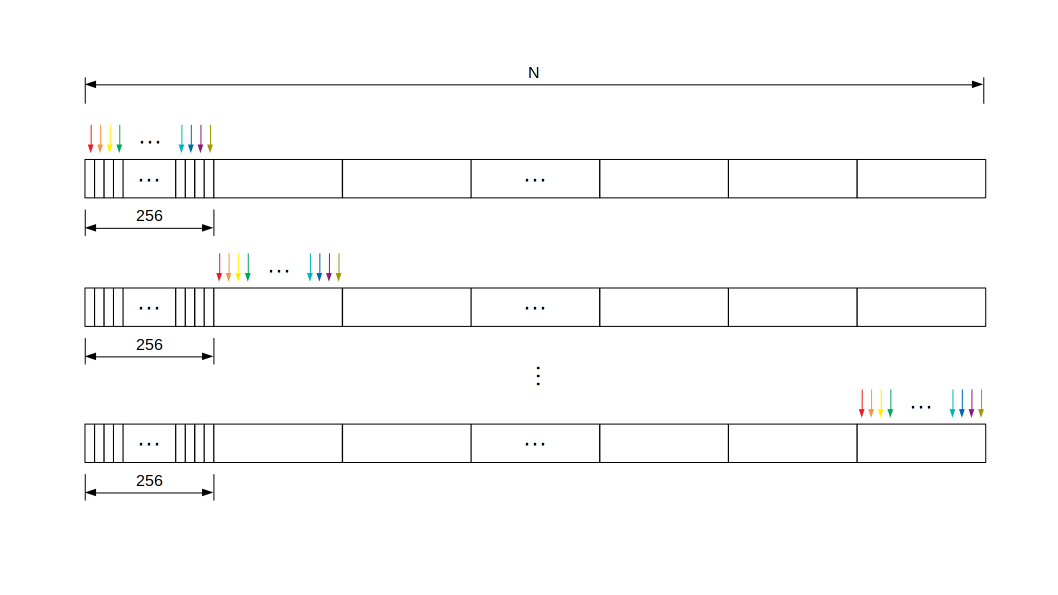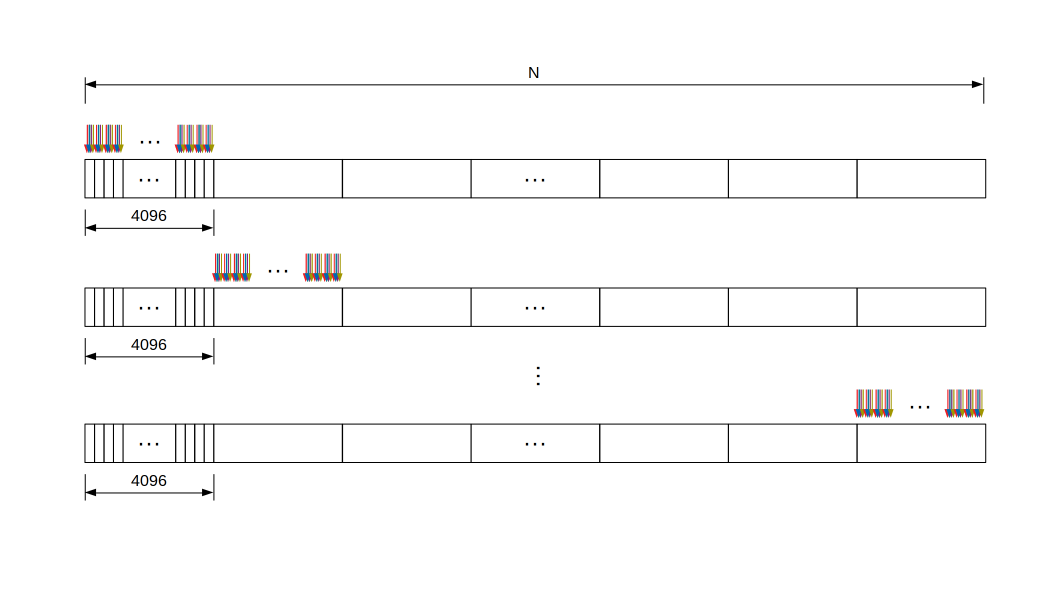# CUDA Block and Grid

## Introduction

I just started to learn CUDA and read this useful blog post “An Even Easier Introduction to CUDA” from NVIDIA. However, I found the images of “Block” and “Grid” in the original blog post was not quite matching with the code in the blog post. So I think I need to express it in a better way.

## Basic Code

This is the piece of CUDA code that I copied from the blog post.

## Block and Grid

I found the figure 1 in the NVIDIA blog post did not quite reflect how the add function was conducted in parallel. So I have made my versions.

### Block

A block consists many threads. In our case, block_dim == block_size == num_threads = 256.In the above figure, each small rectangle is a basic element in the array. When there is only one block, the parallel process could be imagined as block_dim pointers moving asynchronously. That is why you see the index are moving with a stride of block_dim in the following add function when there is only one block.

### Grid

Similarly, a grid consists many blocks. In our case, grid_dim == grid_size = 4096.In the above figure, each small rectangle is a block in the grid. The parallel process could be imagined as block_dim * grid_dim pointers moving asynchronously. That is why you see the index are moving with a stride of block_dim * grid_dim in the following add function.

## Final Remarks

I personally feel it is easier to understand the concept of block and grid with the CUDA code using my figures instead of the one in the original blog post, although that figure was also correct if you think of that a grid wraps a bunch of blocks, a block wraps a bunch of threads, and a thread wraps a bunch of basic array elements.

Lei Mao

03-12-2019

03-12-2019